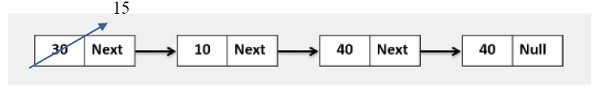# Golang Program to update the node value after the Kth node.

## ExamplesUpdate node after k=10 value node

Approach to solve this problem

Step 1 − Define a method that accepts the head of a linked list.

Step 3 − Iterate the given linked list.

Step 4 − If temp.value is 10, then update temp.next.value=data.

Step 5 − If node value 10 is not found, return the head without updating any node.

## Example

Live Demo

package main
import "fmt"
type Node struct {
value int
next *Node
}
func NewNode(value int, next *Node) *Node{
var n Node
n.value = value
n.next = next
return &n
}
for temp != nil {
fmt.Printf("%d ", temp.value)
temp = temp.next
}
fmt.Println()
}
func UpdateAfterKthNode(head *Node, k, data int) *Node{
// Update after Kth node.
}
for temp != nil{
if temp.value == k{
temp.next.value = data
}
temp = temp.next
}
}
func main(){
head := NewNode(30, NewNode(10, NewNode(40, NewNode(40, nil))))
}
Input Linked list is: 30 10 40 40
Update node after 10th value node, Linked List is: 30 10 15 40